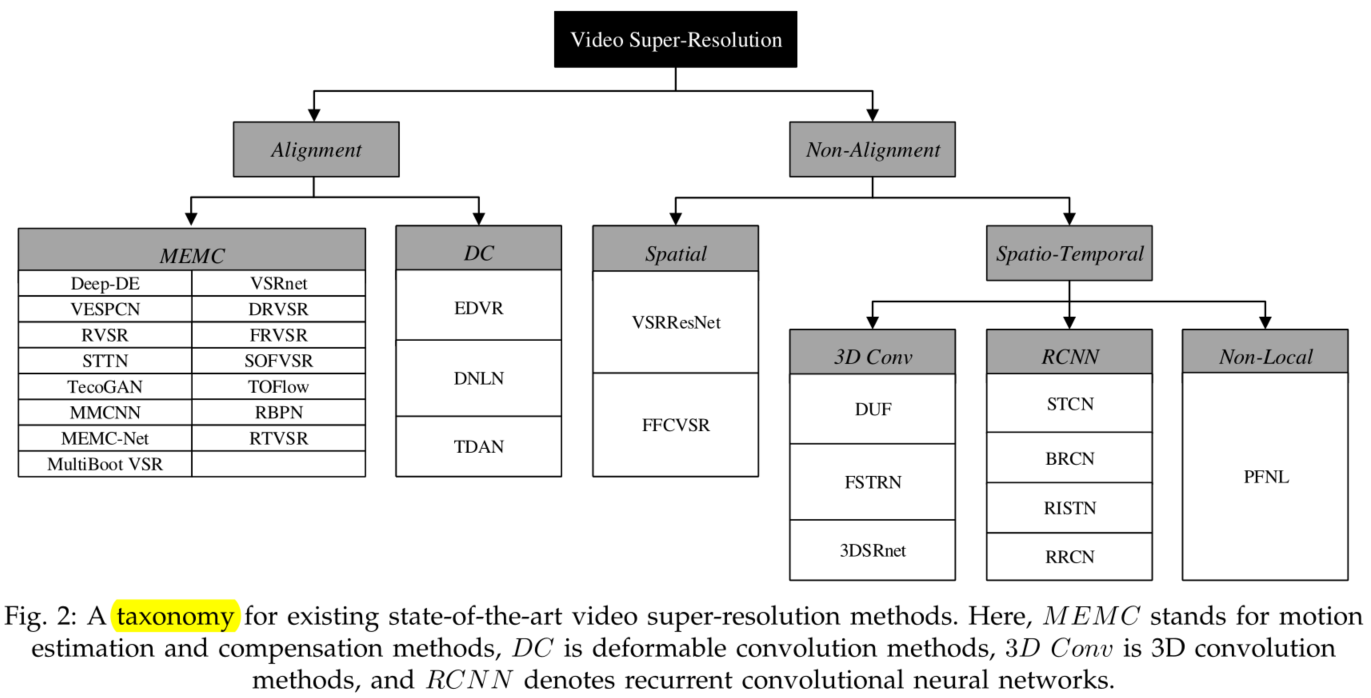# 最新综述丨视频超分辨率研究方法

◎作者档案
Wangsy，一条梦想远大的硕士咸鱼# 看点

1）回顾了基于深度学习的视频超分技术的研究进展；

2）提出了一种基于深度学习的视频超分分类方法，利用不同处理帧间信息的方式进行分类；

3）总结了SOTA方法在一些公共基准数据集上的性能；

4）分析了视频超分任务的一些前景和挑战；

# 背景

### 退化过程

HR的退化过程如下：

$$\hat{I}=\phi\left(I ; \theta_{\alpha}\right)$$

$$\tilde{I}=\phi^{-1}\left(\hat{I} ; \theta_{\beta}\right)$$

$$\hat{I}=(I \otimes k) \downarrow_{s}+n$$

### 评价指标和损失函数

$$\begin{array}{l} \mathrm{PSNR}=10 \log {10}\left(\frac{L^{2}}{\mathrm{MSE}}\right) \ \mathrm{MSE}=\frac{1}{N} \sum {i=1}^{N}(I(i)-\tilde{I}(i))^{2} \end{array}$$

$$\operatorname{SSIM}(I, \tilde{I})=\frac{2 u{I} u{\tilde{I}}+k{1}}{u{I}^{2}+u{\tilde{I}}^{2}+k{1}} \cdot \frac{2 \sigma{I \tilde{I}}+k{2}}{\sigma{I}^{2}+\sigma{\tilde{I}}^{2}+k_{2}}$$

# 总结与分类

### 总结### 分类# 对齐方法

### 运动估计与补偿方法

$$F{i \rightarrow j}=\left(h{i \rightarrow j}, v{i \rightarrow j}\right)=M E\left(I{i}, I{j} ; \theta{M E}\right)$$

$$J=M C\left(I, F ; \theta_{M E}\right)$$

RBPNRBPN采用DBPN作为单图超分网络，ResNet和反卷积作为多图超分网络。使用Vimeo-90K数据集作为训练集，同时使用数据扩充技术。批大小和补丁大小分别设置为8和64×64。$L_1$损失和Adam分别作为损失函数和优化器。初始学习率设置为$10^{−4}$，当总迭代执行一半时，学习率将降低到初始的十分之一。

### 可变形卷积方法EDVR

EDVR是NTIRE19挑战的冠军模型。它提出了两个关键模块：金字塔、级联和可变形对齐模块（PCD）和时空注意融合模块（TSA），分别用于解决视频中的大运动和有效融合多帧。EDVR由PCD、TSA和重建模块三部分组成。首先，通过PCD对输入帧进行对齐，然后通过TSA对对齐的帧进行融合。然后将融合后的结果输入重建模块进行特征提取，再通过上采样得到SR残差图像，将SR残差图像加到直接上采样的目标帧中得到最终的SR帧，结构如下图所示。为了进一步提高性能，EDVR还采用了两阶段的方法，其第二阶段与第一阶段相似，但网络深度较浅。EDVR使用NTIRE19挑战中提出的真实动态场景（REDS）数据集作为训练集。数据集由300个分辨率为720×1280的视频序列组成，每个视频有100帧。作者选取了4个具有代表性的视频（REDS4）作为测试集，其余视频作为训练集进行数据扩充。此外，EDVR采用Charbonnier函数作为损失函数，Adam作为优化器，它以五个连续的帧作为输入。补丁大小和批大小分别设置为64×64和32。初始学习速率设置为$4×10^{−4}$。

# 非对齐方法

### 空间非对齐方法### 三维卷积方法

DUFDUF还提出了一种基于时间轴的视频数据增强方法。通过对不同时间间隔的帧进行顺序或相反顺序的采样，可以得到不同运动速度和方向的视频。在实验中，DUF使用Huber函数作为其损失函数，其中δ=0.01。Adam用作优化器，初始学习速率设置为$10^{-3}$。

### 循环卷积神经网络

RCNN在自然语言、视频、音频等序列数据处理的建模中具有很强的时间依赖性。因此可以使用在视频超分领域中。但是本文没有介绍性能很好的RSDN、RRN等网络，故此处不再做阐述。

### 非局部方法

$$y{i}=\frac{1}{\mathcal{C}(x)} \sum{\forall j} f\left(x{i}, x{j}\right) g\left(x_{j}\right)$$PFNL

PFNL使用非局部残差块来提取时空特征，并提出渐进式融合残差块（PFRB）来进行融合。最后，通过亚像素卷积层的输出加到通过双三次插值上采样的输入帧中，得到SR图像。PFRB由三个卷积层组成。首先，对输入帧进行3×3卷积，串联后通过1×1卷积降低通道维数。并将结果分别与之前的卷积特征图串联，进行3×3卷积。最后的结果被加到每一个输入帧中，得到当前PFRB的输出。此外，为了减少PFRB叠加带来的参数增加，PFNL采用了通道参数共享机制，有效地平衡了参数个数与网络性能之间的权衡。损失函数为Charbonnier函数，使用Adam作为优化器，$10^{−3}$为其初始学习速率。# 性能比较